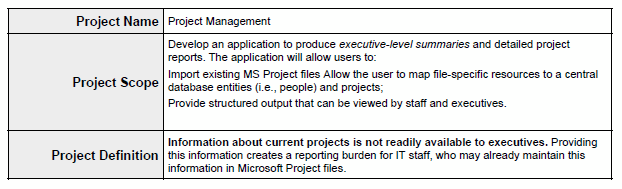## Example Uses of XSL Subtemplates

These are examples of formatting that can be achieved in a report by using XSL subtemplates.

### Handle XML Data with HTML Formatting

If you have XML data that already contains HTML formatting and you want to preserve that formatting in the report, then you can preserve that formatting by using an XSL subtemplate to map the HTML formatting commands to XSL equivalents that can be handled by Publisher.

Note that the HTML must be in XHTML format. This means that all HTML tags must have start and end tags in the data. For example, if the data uses a simple `<BR>` for a break, then you must add the closing `</BR>` before you can use this solution.

Following is some sample data with HTML formatting:

``````<DATA>
<ROW>
<PROJECT_NAME>Project Management</PROJECT_NAME>
<PROJECT_SCOPE>
<p>Develop an application to produce <i>executive-level summaries</i> and detailed project reports. The application will allow users to: </p>
<p>Import existing MS Project files </p>
<p>Allow the user to map file-specific resources to a central database entities (i.e., people) and projects; </p>
<p>Provide structured output that can be viewed by staff and executives. </p>
</PROJECT_SCOPE>
<PROJECT_DEFINITION><b>Information about current projects is not readily available to executives.</b> Providing this information creates a reporting burden for IT staff, who may already maintain this information in Microsoft Project files. </PROJECT_DEFINITION>
</ROW>
</DATA>
``````

Assume a report requirement to display this to retain the formatting supplied by these tags as shown in the following figure:Description of the illustration xdo11g_subt_xsl1.gif

The following subtemplate uses XSL syntax to match the three HTML tags in the XML data. The template then replaces the matched HTML string with its XSLFO equivalent.

``````<xsl:template match="P|p">
<xsl:apply-templates select="text()|*|@*"/>
</fo:block>
</xsl:template>

<xsl:template match="STRONG|B|b">
<fo:inline font-weight="bold">
<xsl:apply-templates/>
</fo:inline>
</xsl:template>

<xsl:template match="EM|I|i">
<fo:inline font-style="italic">
<xsl:apply-templates/>
</fo:inline>
</xsl:template>
``````

To use an XSL syntax:

1. Upload the XSL subtemplate file to the Publisher catalog location: Shared Folders/Projects. Save this subtemplate file as htmlmarkup.xsb.
2. In the main template enter the following to import the subtemplate file:
<?import:xdoxsl:///Projects/htmlmarkup.xsb?>
3. For each field that has HTML markup, call the xsl apply-template command. In this example, there're two fields:
``````<xsl:apply-templates select="PROJECT_SCOPE"/>
<xsl:apply-templates select="PROJECT_DEFINITION"/>``````

The command tells the processor to apply all templates to the value of the element PROJECT_SCOPE and PROJECT_DEFINITION. It then cycles through the subtemplate functions looking for a match.

### Dynamically Apply Formatting to a Portion of Data

This application of subtemplates is useful for documents that require chemical formulae, mathematical calculations, or superscripts and subscripts.

For example, in the sample XML data below CO2 is expected to display as CO2 and H2O is expected to display as H2O.

``````<ROWSET>
<ROW>
<FORMULA>CO2</FORMULA>
</ROW>
<ROW>
<FORMULA>H2O</FORMULA>
</ROW>
</ROWSET>
``````

This can be achieved by using an XSL subtemplate. Using XSL syntax you can define a template with any name, for example, "chemical_formatter" that accepts the FORMULA field as a parameter, and then reads one character at a time. It compares the character with 0 - 9 digits, and if there's a match, then that character is subscripted using the following XSL FO syntax:

``````<fo:inline baseline-shift="sub" font-size="75%">
``````

Here is sample code for the XSL template statement:

``````<xsl:template name="chemical_formatter">
<! -  accepts a parameter e.g. H2O  - >
<xsl:param name="formula"/>
<! -  Takes the first character of the string and tests it to see if it is a number between 0-9 - > <xsl:variable name="each_char"
select="substring(\$formula,1,1)"/>
<xsl:choose>
<xsl:when test="\$each_char='1' or \$each_char='2'
or \$each_char='3' or \$each_char='4' or \$each_char='5'
or \$each_char='6' or \$each_char='7' or \$each_char='8'
or \$each_char='9' or \$each_char='0'">
<! -  if it is numeric it sets the FO subscripting properties  - >
<fo:inline baseline-shift="sub" font-size="75%">
<xsl:value-of select="\$each_char"/>
</fo:inline>
</xsl:when>
<xsl:otherwise>
<! -  otherwise the charater is left as is  - >
<fo:inline baseline-shift="normal">
<xsl:value-of select="\$each_char"/>
</fo:inline>
</xsl:otherwise>
</xsl:choose>
<! -  test if there are other chars in the string, if so the recall the template  - >
<xsl:if test="substring-after(\$formula,\$each_char) !=''">
<xsl:call-template name="chemical_formater">
<xsl:with-param name="formula">
<xsl:value-of select="substring-after(\$formula,\$each_char)"/>
</xsl:with-param>
</xsl:call-template>
</xsl:if>
</xsl:template>
``````
To use this XSL template statement:
1. Save this file as chemical.xsl.
2. Follow the instructions in Upload a Subtemplate. Assume that you name the Sub Template "Chemical" (it's saved as Chemical.xsb) and place it in the following location: `Shared Folders/Subtemplates`.
3. In the main RTF template enter the import syntax:
``````<?import:xdoxsl:///Subtemplates/Chemical.xsb?>
``````
4. To render the XSL code in the report, create a loop over the data and in the VALUE field use:
``````<xsl:call-template name="chemical_formatter">
<xsl:with-param name="formula" select="VALUE"/> </xsl:call-template>
``````

This calls the formatting template with the FORMULA value that is, H2O. Once rendered, the formulae are shown as expected: H2O.